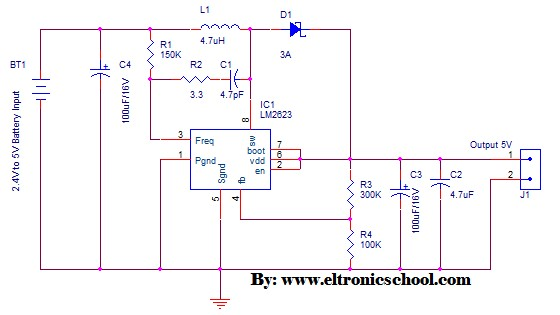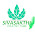Saturday, August 29, 2015

DC to DC Converter 2.4V to 5V Circuit Schematic using LM2623

Eltronicschool. - This is step-up DC to DC converter based on LM2623 IC circuit schematic. With this circuit schematic application, you can produce 5V output using 2.4V input battery. This circuit will suitable when you want to charge your microcontroller system using small voltage.

Please see the DC to DC Converter 2.4V to 5V Circuit Schematic using LM2623 like in Figure 1 below. (You also can read: Circuit Schematic 12V LDO Solar Charge Controller using MOSFET)

Circuit schematicFigure 1. DC to DC Converter 2.4V to 5V Circuit Schematic using LM2623

Component Parts
1. C1 = 4.7pF
2. C2 = 4.7uF
3. C3 and C4 = 100uF/16V
4. R1 = 150K
5. R2 = 3.3 ohm
6. R3 = 300K
7. R4 = 100K
8. D1 = many kinds with I = 3A
9. L1 = 4.7uH
10. IC1 = LM2623 (see sheet data)
Description

Circuit schematic like in Figure 1 above is DC to DC Converter 2.4V to 5V Circuit Schematic using LM2623. This step-up DC to DC converter based on LM2623 IC. LM2623 is The LM2623 is a high-efficiency, general-purpose step-up DC-DC switching regulator for battery-powered and low input voltage systems. It accepts an input voltage between 0.8 V and 14 V and converts it into a regulated output voltage between 1.24 V and 14 V. Efficiencies up to 90% are achievable with the LM2623.

In order to adapt to a number of applications, the LM2623 allows the designer to vary the output voltage, the operating frequency (300 kHz to 2 MHz) and duty cycle (17% to 90%) to optimize the part's performance. The selected values can be fixed or can vary with battery voltage or input to output voltage ratio. The LM2623 uses a very simple, on/off regulation mode to produce good efficiency and stable operation over a wide operating range. It normally regulates by skipping switching cycles when it reaches the regulation limit (Pulse Frequency Modulation).

Thank you for your coming here in www.eltronicschool.com site, we hope the article above will help you to know more about your an electronic circuit design and software in this time, etc. Please comment here when you want to share and other. Thank you.

1 comment:

1.what is the output A